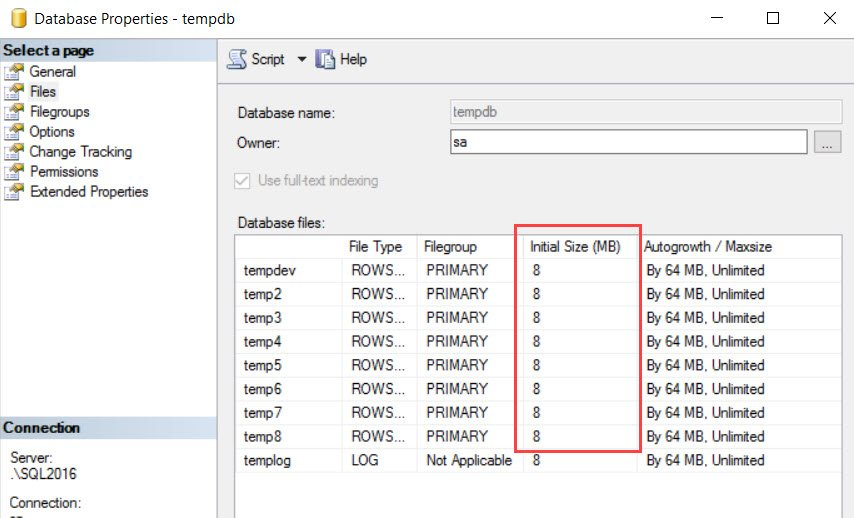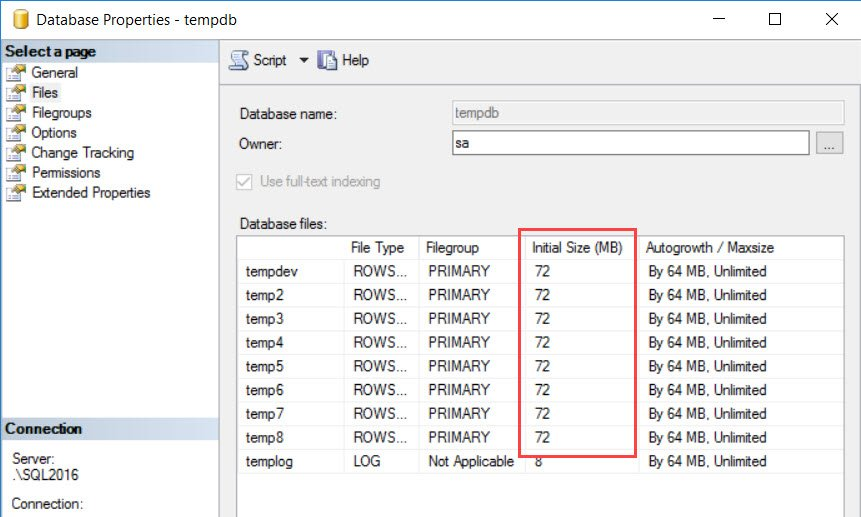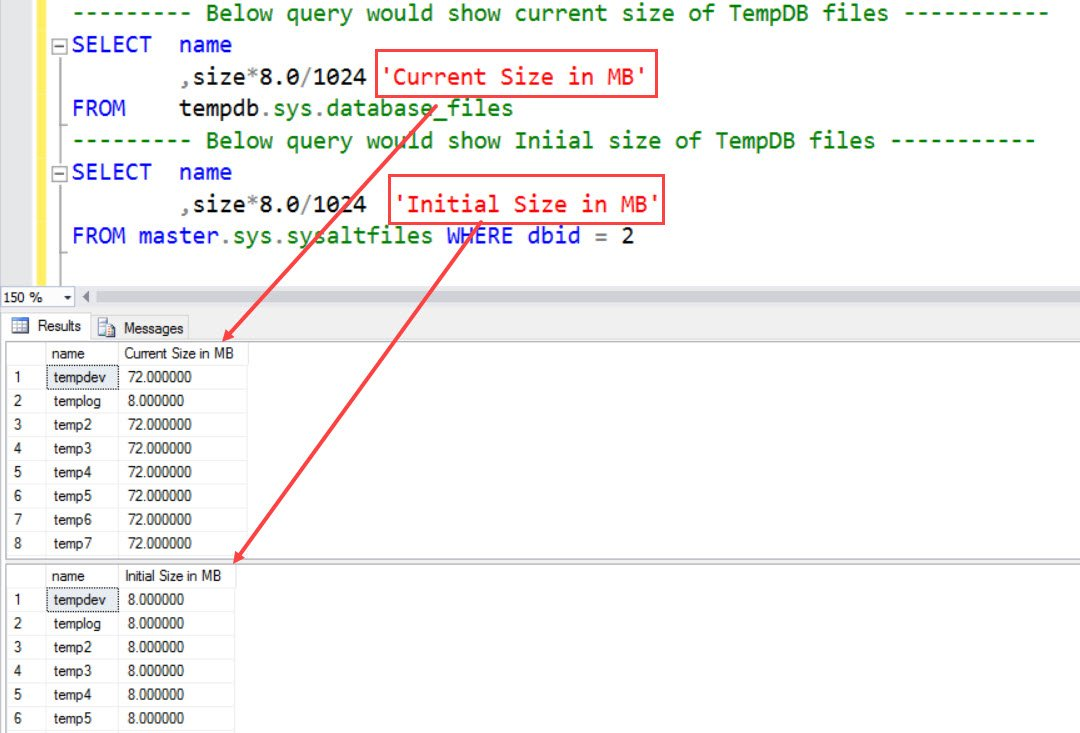# What is the Initial Size of TempDB? – Interview Question of the Week #120

Question: What is the Initial Size of TempDB? Trust me, this question is way more complicated than you read in one line.

Answer: It is well known fact that TempDB is a special system database in SQL Server, which is recreated every time the SQL Server process is started. This database file can grow, if allowed, based on usage of the database. When the SQL process restarts, the size would come back to original size.

If any modification of Temp DB files is done by a DBA, they would be retained.

Let’s do this small test to understand it. I am using SQL Server 2016 so your result might vary if you are using older SQL version. After restart of SQL Server, here is how Temp DB looks like.Now, let’s insert some rows in a table in Temp database. Here is the simplest possible script.

```SET NOCOUNT ON
GO
USE tempdb
GO
CREATE TABLE #temp (a CHAR(8000))
GO
INSERT INTO #temp VALUES ('a')
GO 50000
```

As soon as above loop is complete, here is the file details.As we can see above Initial size is shown as 72 MB, which is incorrect. It’s showing the current size. So, if we restart SQL it would not the 72 MB but it would be 8 MB (as shown in earlier snapshot)

Now, the question is – how do we know the size of tempdb database files after restart?

## WORKAROUND/SOLUTION

Here are the queries which we can use to find initial size and current size.

```--------- Below query would show current size of TempDB files -----------
SELECT	name
,size*8.0/1024 'Current Size in MB'
FROM	tempdb.sys.database_files
--------- Below query would show Iniial size of TempDB files -----------
SELECT	name
,size*8.0/1024  'Initial Size in MB'
FROM master.sys.sysaltfiles WHERE dbid = 2
```So, now you can identify the size of the Temp DB after restart. Simple but powerful!

Reference: Pinal Dave (http://blog.SQLAuthority.com)